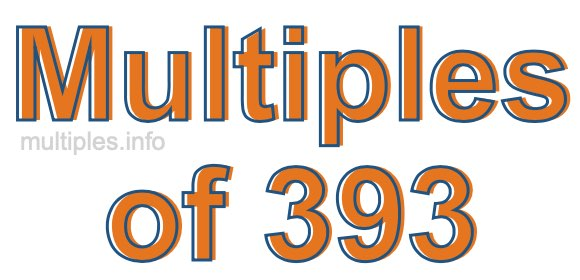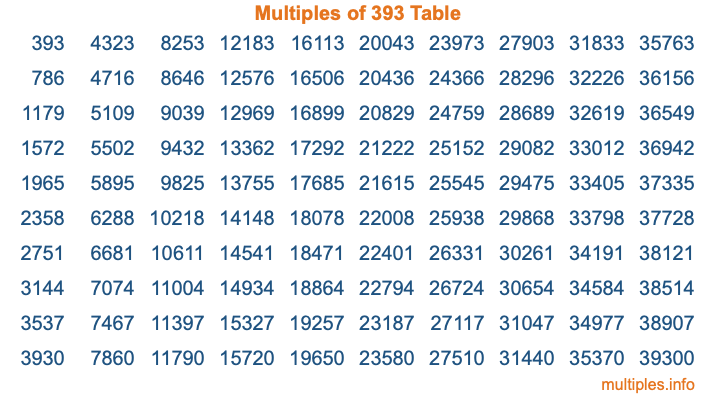Multiples of 393Welcome to the Multiples of 393 page. Here we will first teach you everything you will ever need to know about the multiples of 393, and then give you a study guide summary of everything we taught you to make sure you remember it all. Use this page to look up facts and learn information about the multiples of 393. This page will make you a multiples of three hundred ninety-three expert!

Definition of Multiples of 393
Multiples of 393 are all the numbers that when divided by 393 equal an integer. Each of the multiples of 393 are called a multiple. A multiple of 393 is created by multiplying 393 by an integer.

Therefore, to create a list of multiples of 393, you start with 1 multiplied by 393, then 2 multiplied by 393, then 3 multiplied by 393, and so on for as long as you want. Thus, the list of the first five multiples of 393 is 393, 786, 1179, 1572, and 1965. To see a larger list of multiples of 393, see the printable image of Multiples of 393 further down on this page. We also have a category where you can choose any nth multiple of 393.

Multiples of 393 Checker
The Multiples of 393 Checker below checks to see if any number of your choice is a multiple of 393. In other words, it checks to see if there is any number (integer) that when multiplied by 393 will equal your number. To do that, we divide your number by 393. If the the quotient is an integer, then your number is a multiple of 393.

Is  a multiple of 393?

Least Common Multiple of 393 and ...
A Least Common Multiple (LCM) is the lowest multiple that two or more numbers have in common. This is also called the smallest common multiple or lowest common multiple and is useful to know when you are adding our subtracting fractions. Enter one or more numbers below (393 is already entered) to find the LCM.

Check out our LCM Calculator if you need more details about the Least Common Multiple or if you need the LCM for different numbers for adding and subtraction fractions.

nth Multiple of 393
As we stated above, 393 is the first multiple of 393, 786 is the second multiple of 393, 1179 is the third multiple of 393, and so on. Enter a number below to find the nth multiple of 393.

th multiple of 393

Multiples of 393 vs Factors of 393
393 is a multiple of 393 and a factor of 393, but that is where the similarities end. All postive multiples of 393 are 393 or greater than 393. All positive factors of 393 are 393 or less than 393.

Below is the beginning list of multiples of 393 and the factors of 393 so you can compare:

Multiples of 393: 393, 786, 1179, 1572, 1965, etc.

Factors of 393: 1, 3, 131, 393

As you can see, the multiples of 393 are all the numbers that you can divide by 393 to get a whole number. The factors of 393, on the other hand, are all the whole numbers that you can multiply by another whole number to get 393.

It's also interesting to note that if a number (x) is a factor of 393, then 393 will also be a multiple of that number (x).

Multiples of 393 vs Divisors of 393
The divisors of 393 are all the integers that 393 can be divided by evenly. Below is a list of the divisors of 393.

Divisors of 393: 1, 3, 131, 393

The interesting thing to note here is that if you take any multiple of 393 and divide it by a divisor of 393, you will see that the quotient is an integer.

Multiples of 393 Table
Below is an image of the first 100 multiples of 393 in a table. The table is in chronological order, column by column. The first column has the first ten multiples of 393, the second column has the next ten multiples of 393, and so on.The Multiples of 393 Table is also referred to as the 393 Times Table or Times Table of 393. You are welcome to print out our table for your studies.

Negative Multiples of 393
Although not often discussed or needed in math, it is worth mentioning that you can make a list of negative multiples of 393 by multiplying 393 by -1, then by -2, then by -3, and so on, to get the following list of negative multiples of 393:

-393, -786, -1179, -1572, -1965, etc.

Multiples of 393 Summary
Below is a summary of important Multiples of 393 facts that we have discussed on this page. To retain the knowledge on this page, we recommend that you read through the summary and explain to yourself or a study partner why they hold true.

There are an infinite number of multiples of 393.

A multiple of 393 divided by 393 will equal a whole number.

393 divided by a factor of 393 equals a divisor of 393.

The nth multiple of 393 is n times 393.

The largest factor of 393 is equal to the first positive multiple of 393.

393 is a multiple of every factor of 393.

393 is a multiple of 393.

A multiple of 393 divided by a divisor of 393 equals an integer.

393 divided by a divisor of 393 equals a factor of 393.

Any integer times 393 will equal a multiple of 393.

Multiples of a Number
Here you can get the multiples of another number, all with the same attention to detail as we did for multiples of 393 on this page.

Multiples of
Multiples of 394
Did you find our page about multiples of three hundred ninety-three educational? Do you want more knowledge? Check out the multiples of the next number on our list!